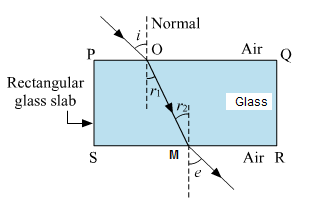# (i) If the angle of incidence and angle of emergence of a light ray falling on a glass slab are i and e respectively, prove that, i = e.

more_vert

(i) If the angle of incidence and angle of emergence of a light ray falling on a glass slab are i and e respectively, prove that, i = e.

more_vert

verifiedIn the fig SR || PQ and OM is the reflected ray Hence r1=r2

Let μ be the refractive index of the glass slab.

Then, according to Snell's law,

Then, according to Snell's law,

$$\frac{sin\,\,i}{sin\,\, r_1}$$ = μ .....(i)

and

$$\frac{sin\,\,r_2}{sin\,\, e}$$ = $$\frac{1}{μ}$$ .... .....(ii)

But r1=r2

Putting (iii) in (i), we get

$$\frac{sin\,\,i}{sin\,\, r_2}$$ = μ .....(iv)

Multiplying (ii) and (iv), we have

$$\frac{sin\,\,i}{sin\,\, r_2}$$ x $$\frac{sin\,\,r_2}{sin\,\, e}$$ = μ x $$\frac{1}{μ}$$

∴ $$\frac{sin\,\,i}{sin\,\, e}$$ = 1

∴ i = e proved